# Math in Focus Grade 3 Cumulative Review Chapters 8 and 9 Answer Key

Practice the problems of Math in Focus Grade 3 Workbook Answer Key Cumulative Review Chapters 8 and 9 to score better marks in the exam.

## Math in Focus Grade 3 Cumulative Review Chapters 8 and 9 Answer Key

Concepts and Skills

Complete. Use the digits 1, 3, and 8. (Lesson 8.3)

Question 1.
Write two 3-digit even numbers.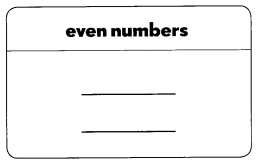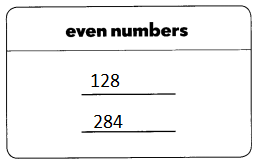Explanation:
a whole number that is able to be divided by two into two equal whole numbers
The numbers 0, 2, 4, 6, and 8 are even numbers.

Question 2.
Write four 3-digit odd numbers.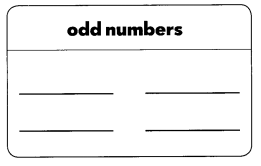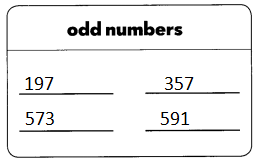Explanation:
An odd number is a number that cannot be divided into two equal groups.
Even numbers end in 2, 4, 6, 8 and 0 regardless of how many digits they have
Odd numbers end in 1, 3, 5, 7, 9.

Fill in the blanks. Use mental math. (Lesson 8.1)

Question 3.
6 ÷ 2 = ___
60 ÷ 2 = ___ tens ÷ 2
= ___ tens
= ____
600 ÷ 2 = hundreds ÷ 2
= ____ hundreds
= _____
6 ÷ 2 = 3
60 ÷ 2 = 30 ; 3 tens ÷ 2
= 1 tens and 5 ones
= 15
600 ÷ 2 = 3 hundreds ÷ 2
= 1 hundreds 5 tens and 0 ones
= 150

Divide.

Question 4.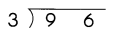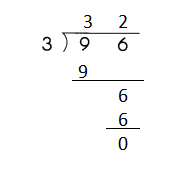Explanation:
96 ÷  3 = 32
The quotient is 32
the remainder is 0

Question 5.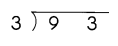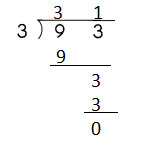Explanation:
93 ÷  3 = 31
The quotient is 31
the remainder is 0

Question 6.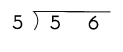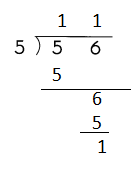Explanation:
56 ÷  5 = 11
The quotient is 11
the remainder is  1

Question 7.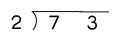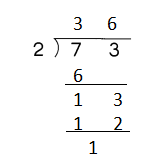Explanation:
73 ÷ 2 = 36
The quotient is 36
the remainder is 1

Question 8.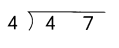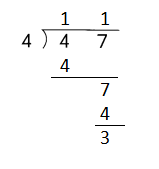Explanation:
47 ÷  4 = 11
The quotient is 11
the remainder is 3

Question 9.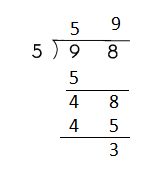Explanation:
98 ÷  5 = 59
The quotient is 59
the remainder is 3

Divide. Then match the division facts that have the same quotients. (Lessons 8.1 and 8.2)

Question 10.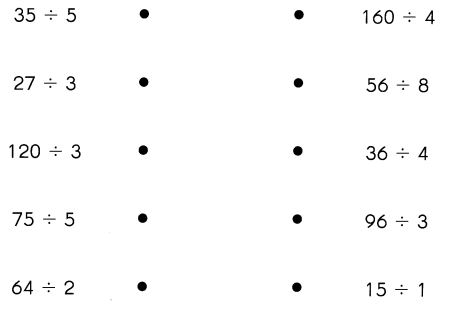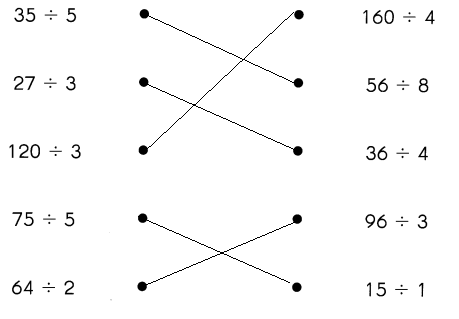Explanation:
The value of quotients are matched
35÷  5 = 7
27÷  3 = 9
120 ÷  3 = 40
75 ÷  5 = 15
64 ÷  2 = 32
160 ÷  4 = 40
56 ÷  8 = 7
36 ÷  4 = 9
96 ÷  3 = 32
15 ÷  1 = 15

Problem Solving

Question 11.
Roland has 125 trading cards. Ian has three times as many trading cards as Roland. How many trading cards does Ian have?
375 cards
Explanation:
Ian has three times as many trading cards as Roland.
125 x 3 = 375
375 trading cards that Ian have

Question 12.
Mr. Hansen gives out 250 pencils to 5 classes. Each class receives an equal number of pencils. How many pencils does each class receive?
50 pencils
Explanation:
Mr. Hansen gives out 250 pencils to 5 classes.
Each class receives an equal number of pencils.
250 ÷   5 = 50
50 pencils that each class receive

Question 13.
Mike is three times as tall as Pamela. Pamela is 2 feet tall.

a. How tall is Mike?
2 x 3 = 6 feet
Explanation:
Mike is three times as tall as Pamela.
Pamela is 2 feet tall.
2 x3 = 6 feet

b. How many feet taller is Mike than Pamela?
6 – 2 = 4
Explanation:
Mike is three times as tall as Pamela.
Pamela is 2 feet tall.
2 x 3 = 6 feet
6 – 2 = 4

Question 14.
Mrs. Herra buys 3 boxes of oranges. She also buys 6 boxes of apples. Each box contains 65 pieces of fruit.

a. How many boxes of fruit does Mrs. Herra buy?
3 x 6 = 18 boxes
Explanation:
Mrs. Herra buys 3 boxes of oranges.
She also buys 6 boxes of apples.
3 x 6 = 18

b. How many pieces of fruit does Mrs. Herra buy?
585 pieces of fruit that Mrs. Herra buy
Explanation:
Mrs. Herra buys 3 boxes of oranges.
oranges 3 x 65 = 195
She also buys 6 boxes of apples.
apples 6 x 65 = 390
390 + 195 = 585 fruits

Question 15.
Sha un has 96 ounces of lemonade. He pours all the lemonade equally into 6 jugs. He then pours out one of the jugs of lemonade to fill 2 glasses. How many ounces of lemonade does each glass hold?
Explanation:
Sha un has 96 ounces of lemonade.
He pours all the lemonade equally into 6 jugs.
96 ÷ 6 = 16
He then pours out one of the jugs of lemonade to fill 2 glasses.
16 ÷ 2 = 8
8 ounces of lemonade that each glass hold

Question 16.
A watch costs $56. A camera costs double the amount of the watch. How much do the two items cost in all? Answer:$112 items cost
Explanation:
A watch costs \$56.
A camera costs double the amount of the watch.
56 x 2 = 112
112 that the two items cost in all

Question 17.
Ben has 4 cartons of crayons. Each carton contains 1 20 crayons. He puts them equally into 6 cartons. How many crayons are in each carton?
80 crayons
Explanation:
Ben has 4 cartons of crayons.
Each carton contains 1 20 crayons.
4 x 120 = 480
He puts them equally into 6 cartons.
480 ÷  80
80 crayons are in each carton

Question 18.
Natalie has 275 centimeters of ribbon to make headbands. She makes 4 headbands and has 15 centimeters of ribbon left. What length of ribbon does she use for each headband?